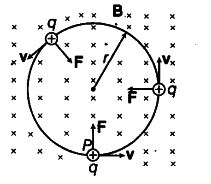# A particle of charge q and mass m is moving with velocity v

A particle of charge q and mass m is moving with velocity v. It is subjected to a uniform magnetic field B directed perpendicular to its velocity. Show that, it describes a circular path. Write the expression for its radius. Foreign 2012 Sol. A charge q projected perpendicular to the uniform magnetic field B with velocity v. The perpendicular force, F = q(v X B), acts like a centripetal force perpendicular to the magnetic field. Then, the path followed by charge is circular as shown in the figure.The Lorentz magnetic force acts as centripetal force, thus qvB = m\${{v}^{2}}\$ / r
Here, r = radius of the circular path followed by charge projected perpendicular to the uniform magnetic field.